# A gentle introduction to Technical Analysis.

Have you ever seen one of those complicated charts with so many lines and shapes that seemed too fancy and some sort of rocket science? Well, it turns out that these are actually the simplest means to analyze markets. Anyone can understand the displays given several minutes of explanations, but the trick is, how to use them properly? and how to develop new strategies? Technical analysis in layman’s terms is when you look at a chart, add some lines and calculations to it and try to project the future direction of the price, it is what retail brokers are promoting in their marketing techniques. It assumes that all you need for the future is historical data. Among other assumptions, technical analysis suggests that markets are not efficient (thus the ability to project the future from the past). The market efficiency hypothesis is the technical analyst’s greatest enemy as one of its principles is that in the weak form of efficiency, you cannot earn excess returns from technical analysis. Hence, technical analysis gets shot down at the very first level, and in the second level, fundamental analysis gets its share of the beating. It is fair to assume that at some point in the future, markets have no choice but to be efficient, let’s just hope now that we have enough time to take advantage of the fact that we are still able to use some techniques that enable us to extract some decent returns, and if we’re really good, more than decent returns, otherwise known as alpha.

Calculating autocorrelations of price returns in order to check whether there is a form of predictability shows that most financial returns data exhibit no significant autocorrelation (also called serial correlation), which in turn means that the past values have no predictive power. This theory is highly debatable and has many valid points but for us, alpha seekers, we are not very concerned with it. We remain focused on generating strategies, back-testing them in a correct way, and constantly updating them. The three main principles of Technical analysis are:

• History tends to repeat itself.
• The market discounts everything.
• Prices move in trends.

It mainly originated from Japan, although it is also stated that it came from Amsterdam. With that being said, let us discover this wonderful must-have skill.

# CHARTING ANALYSIS

The asset’s direction on a timeline axis can be threefold:

• Uptrend: prices are making higher highs.
• Downtrend: prices are making lower lows.
• Range: prices fluctuate around the same level for extended periods of time.

Charting is the most basic technical tool. It relies on psychological levels that certain buyers observe carefully. Prices tend to stall while reaching a resistance level and can reverse afterwards. A support level is where a falling price may rebound on and eventually goes upwards. A trend line can be trending up or down and is the same as a support or a resistance except that it is not perfectly horizontal. Two trend lines engulfing the price chart (one from the below and one from the top) are referred to as a channel which can be up-trending or down-trending. These tools, if mastered, can be extremely powerful. It is worth noting that these levels work better at higher time intervals, for example a resistance level at an hourly time frame might work much better if on a daily time frame that same level is also acting as a resistance. A decent framework is to start through a top-down approach by charting on a monthly, then weekly, and finally daily time frame and see whether they confirm each other or not. A red flag should be raised when on a weekly level you find a support zone while simultaneously it is acting as a resistance level on a daily time frame. In that case, either adjust your trading horizon or wait for better confirmation. Acting on the levels should only be done with a lot of conviction and not just a hunch that the weekly may overwhelm the daily time frame.

Finding support and resistance levels can be of many ways:

• Graphical: Simply tracing at least three points together gives you a support or resistance level.
• Fibonacci: Retracements and projections are heavily used through Fibonacci retracements.
• Pivot points: The simplest and most objective method is to calculate the support and resistance levels through Pivot points.

The below figure shows a support zone on the EURUSD pair. The 1.05 level has been acting as a very obvious and powerful support zone through empirical evidence and psychological observation. Regarding the second reason, some levels can be inferred with an adequate confidence interval if they are well-rounded. For example, an asset that trades between \$70 — \$120 might have something going on at exactly \$100. It can be a powerful level of either support or resistance (depending on the position of the price relative to it), also a currency pair that trades between the range of 0.990 and 1.100 will probably have traders positioned at exactly 1.000.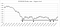A support level on EURUSD which clearly shows a tradable zone around 1.05. The zone is as technical as it is psychological. A level that has been respected at least three times is considered a strong level and has a high probability of changing the course of the price when encountered again.

The following figure shows a resistance zone on the same chart. The psychological and empirically evidenced level of 1.15 is acting as a good resistance level. Each time the price approaches that level, it stalls or reverses course completely.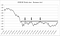Resistance level on EURUSD. As with the previous chart, the 1.15 level is also psychological and can be apparent to many market participants which adds further conviction to its success probability.

The next figure shows a channel (a price surrounded by two trend lines). This is the equivalent of having both a tilted support and a resistance level that engulf the price. Notice how the price has been contained for a period of time inside the channel. A powerful trading strategy would be to search for a double confirmation from a horizontal support/resistance as well as a reversal level from a channel.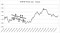Price channel on EURUSD that has been broken to the downside and provided a brief short opportunity before fundamentals most likely took the lead and pushed the trend upwards. Of course, even technically speaking, the 1.05 level is considered a major support zone.

# LINES AND CANDLESTICKS

The two most common charts are line charts and candlestick charts. Both should be used together. We will omit discussions on bar charts due to their less-important features when compared to candlesticks. For example, when we are tracing supports and resistances, it is better to use line charts to accurately detect the closing prices that touch the lines (levels). After having done that, switching to a candlestick chart is a must, in order to understand the market behavior and search for candlestick patterns that could confirm or defer our views. Line charts are intuitive, it is what we get when we draw lines from the closing prices’ points in the time axis.

Candlesticks are a practical and easy way to understand the sentiment and the state of the market. Four basic information can be found when looking at a candle, the open price, the closing price, the high, and the low of the day (or week, month, etc.). Below is a simple representation of a bullish and a bearish candle.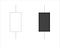Bullish candle in white and a bearish candle in black. The sticks on the above are the highs and the sticks from the below are the lows of the period.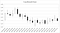Candlestick chart.

There are many candlesticks patterns that are used to confirm a bias. I’ve discussed them in a prior article here on Medium: Do candlestick patterns work on their own? A systematic FX back-test using Python.

# MOVING AVERAGES

One of the very first things we should do is identify the trend. Visually, it can be easy and by practice it will get easier but there is also a way to quantify this view. Moving averages help us determine the trend of the asset and to time our re-entries. How they work is pretty simple, imagine you have 20 datasets and you want to calculate a 4-period moving average. The way you do this is by simply taking the average of the first 4 values, then you will ditch the first value and calculate the average of the second value until the fifth value (hence, a 4-period average), and so on and so forth. The concept is pretty simple and is very helpful for certain trading strategies, especially automated ones. The major drawback of moving averages is that they are lagging, because they smooth out prices, they tend to recognize reversals a bit late. This is the same as forecasting the past and therefore not very helpful when used alone.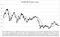EURUSD chart with moving averages. When the short-term moving average (light grey) crosses the long-term moving average (dark grey) from the below, it is referred to as a golden cross and signals a bullish change. The other way around is called a dead cross and signals a bearish shift.

Another type of moving averages is the exponential moving average. It is generally better-suited for trading and has less lag as it gives more weight to the last values and thus emphasizing the present at the expense of the far past. It is up to the trader to decide which one fits his or her style although the simple moving average is the most common one. The two do not differ by much but sometimes they are the edge between buying and selling. They nevertheless, remain lagging indicators. They are also used for position accumulation, for instance, if you are long an asset, and it keeps going up until at some point it reverts back to test its moving average or a new support zone (after having been a resistance before being broken by the uptrend), then it may be a good buy re-entry point if you are still convinced by your long bias.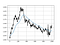From left to right: 200-Day simple moving average, 200-Day exponential moving average.

Two moving averages can be used together to form a more adequate strategy. If the short-term moving average crosses the long-term moving average from the below, it can be seen as a buy signal and the beginning of an uptrend. However, moving averages are terrible in ranging markets, they provide no useful information as prices go right through them.

To learn more about how to code moving averages in Python, check out this article I wrote here in Medium: How to code different types of moving averages in Python.

# REVERSAL & CONTINUATION PATTERNS

The charts sometimes display patterns that repeat themselves throughout time and have a fairly predictable outcome. They can be reversal or continuation patterns:

• Reversal patterns: Head and shoulders, inverted head and shoulders, double top, triple top, double bottom, triple bottom, etc.
• Continuation patterns: Flags, rectangles, ascending triangles, descending triangles, etc.

Probably, the most famous ones are the double figures and the head and shoulders pattern. Generally, they work better at higher time frames (e.g. daily and weekly) but they can also be predictive in shorter time frames.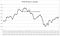Head and shoulders. A very obvious figure that has delivered on its promise. The three twisted lines represent a shoulder, head, and another shoulder (from left to right). Notice how a tiny head and shoulders pattern also exists inside the head of the big pattern.

With reversal patterns, the optimal way to trade them is when prices pull-back to the level that has just been broken which is also called the neck of the figure. Directly entering the trade after the breakout can be a bit dangerous albeit not wrong. The head and shoulders figure is without a doubt the most popular pattern in the financial markets and the fact that it is, makes it even more noticed by traders (professional and retail) thus making it a self-fulfilling prophecy. Other head and shoulders (and inverse head and shoulders) patterns can be less obvious and that might hurt their success rate. Traders are encouraged to act on the apparent ones so that they make sure everyone sees it and interpret it the same way.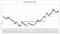Triple bottom. It is characterized by having three bottoms (tops) on approximately the same level.

The neck of a reversal pattern is the lowest or highest point (depending on the bullishness or bearishness) inside the figure. For example, in the above chart, the highest point inside the figure was around 0.95 which is why we have drawn a line and projected it to form a neck that has to be broken, pulled-back to, and then the trend will probably reverse to the upside.

The rectangle pattern can be less obvious than the head and shoulders and the double top (bottom) figures but can deliver a confirmation to an already established trend. The precise definition of the rectangle is a short-term horizontal channel, as opposed to the slightly tilted channels we are used to. From that, we should also know that flag patterns are also tilted channels but in a short-term period. A channel may run for several weeks on a daily chart while a flag may run for several days. Both rectangles and flags are continuation patterns and suggest that the trend will continue. In a downward trending market, the lower level must be broken so the flag pattern gets validated. Again, these minor figures are used to confirm a slightly established bearish and cannot really be used to form a trade opinion. We will always stress that technical analysis must be used as a secondary analysis that comes after fundamental analysis, albeit impossible in the very-short term (e.g. minutes and hours).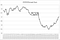The rectangle pattern occurs during periods of congestion after an uptrend or a downtrend. It has a similarity to the flag pattern.

# TECHNICAL INDICATORS

They are price-derived indicators that use formulas to translate the momentum of price levels into quantifiable time-series. The most famous three are the relative strength index (RSI), Moving average convergence-divergence (MACD) indicator, and the stochastic oscillator. These indicators are lagging but carry very useful information. They are used for mainly two purposes:

• Determine the levels of overbought and oversold zones (exception: MACD).
• Show that prices are losing their strength through a divergence manifestation.

Other less-known indicators include the ADX, momentum indicator, CCI, and the OBV, however we will only discuss the three popular ones. We stress that an oversold / overbought position is not a trade trigger. Prices can remain in these levels for a long time. Divergences have to be confirmed by a resistance or a support level as well and they tend to be early signals that can leave many traders with a large negative position.

The two indicators that are bounded and can have overbought and oversold zones are the RSI and the stochastic oscillator. The MACD is unbounded and can only be used through divergence analysis (it works better on trending markets). The indicators have default parameters that are the most used and it is best left untouched, as with trading, one is better-off looking at a pattern that everyone can see and act on, otherwise, it is useless to force patterns out of nothing. The RSI’s oversold zone is 30 and its overbought zone is 70 while the stochastic’s oversold zone is 20 and its overbought zone is 80. Both indicators have a default look-back period of 14, that means they use the last 14 values to calculate their values. The stochastic indicator also has another well-used look-back period, 5 periods. Traders can use both simultaneously (14 and 5) to confirm trends or correction (as we will see later in the chapter 3).

The MACD indicator is also useful although it is lacking one prediction element, that is, overbought and oversold zones. This leaves it with just divergence analysis. The below figure shows the EURUSD daily values with the three indicators for the same period. RSI (14), MACD (26, 12, 9), and Stochastics (14).

If you want to know more about the RSI and how to use it properly, I’ve writtenan article about it here in Medium: Creating a Trading Strategy Using the RSI & the Bollinger Bands. New Ideas Using Python.

# BOLLINGER BANDS

Before we talk about a very important technical indicator called the Bollinger Bands, let’s explore some basic descriptive statistics concepts. The term statistics englobes many sub-fields, notably descriptive statistics and inferential statistics. Below are the descriptive statistical properties that interest us.

What is the mean? It is a measure of the central tendency and it is our best guess for the expected value. For example, if we have the following list X = (1, 2, 3, 4, 5) then the mean is 3 as per the intuitive formula below:

What is the median? It is the value in the middle of the ordered dataset (position). In this case it will be the number 3 even if the list is (2, 3, 1, 5, 4). To be more complete, the full formula for the median of an odd number list is given by:

What is the mode? It is the value that is repeated the most. In this case, it does not exist as each number has only appeared once. The mode is unlikely to exist when it comes to financial returns data. Imagine a dataset composed of 500 returns data, how many times do you think the value 0.5413975% will repeat itself? Highly unlikely.

Dispersion measures how much the data is away from the mean on average. They tell us how much our dataset is spread around. The more a dataset is dispersed, the more volatile and riskier it is. Volatility (standard deviation) is a key concept in finance. Variance is the squared deviations from the mean, we square it so as to force the distance to be non-negative, finally we take the square root to make the measure have the same units as the mean, in a way we are comparing apples to apples (standard deviation). Variance is calculated through this formula:

Following our logic, standard deviation is therefore:

In the case our list is a sample (and not a population) we divide by the unbiased estimate N — 1 (else, if it is a population, we divide by N). With financial data we are almost always dealing with samples. Now it is time to understand the concept of a normal distribution. This bell-shaped figure is the essence of statistics and the foundation of the statistical theory. It has the following characteristics:

• Approximately 68% of the data falls within one standard deviation of the mean.
• Approximately 95% of the data falls within two standard deviations of the mean.
• Approximately 99% of the data falls within three standard deviations of the mean.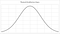A normal distribution. In the center lies the mean, median, and mode. Its symmetric shape produces the equality of the three statistical moments described.

Two other measures of distribution that are worth mentioning:

• Skewness: A measure of the asymmetry of a dataset around its mean. The normal distribution has a skewness of zero. Financial returns do not have this property and are typically positively or negatively skewed.
• Kurtosis: A measure of how fat the distribution’s tails are. The normal distribution has a kurtosis of 3. However, the formula may be modified so as to make it a uniform zero, and in this case, the measure is called excess-kurtosis. Financial data typically have fatter tails than usual.

Using the list (1, 2, 3, 4, 5), we can calculate different dispersion measures without resorting to calculators. Variance can be calculated assuming the list is a sample. When dealing with financial data, we prefer to always use a sampling method and therefore we divide by N — 1, in this case it is 4.

(1–3)^2 = 4

(2–3)^2 = 1

(3–3)^2 = 0

(4–3)^2 = 1

(5–3)^2 = 4

σ^2 = = 2.5

σ is therefore the square root of 2.5 or approximately 1.58, hence, 68% of the time, the value we get deviates from the mean by about 1.58. In other words, on a dataset of 100 elements, we expect that 68 of them will have a value between (1.42, 4.58) and 95 of them will have a value between (-0.16, 6.16) which in this case seems to be 100% of the time because we know the full dataset and do not expect new items, but in real life we do not know everything, we do not even know the distribution the variables follow.

A hint, financial data do not follow a normal distribution, but for modelling and simplicity reasons, we assume that they do. Now it is time to discuss the Bollinger Bands. The concept is really simple, by default, the indicator calculates a 20-period simple moving average and two standard deviations away from the prices, and plot them alongside the price to get a better understanding of where prices are. Clearly, the below chart seems easy to understand, every time the price reaches one of the bands (upper band being the mean added to two multiplied by the standard deviation), a contrarian position is most suited. I am more of a fan of the Bollinger(60, 3) which means 3 standard deviations of the 60-period moving average. Notice how the bands act as supports and resistances. The Bollinger bands work much better in ranging markets.

One last word before we finish, we have to talk about the role of correlation in any statistical analysis. Correlation (ρ) measures the degree of the linear relationship between variables, it has the following features:

• ρ = -1, means a perfectly negative relationship. They move in the opposite direction.
• ρ = 0, means there is no linear relationship between the two.
• ρ = 1, means a perfect linear relationship between the datasets. They move hand in hand.

Correlation does not imply causality. It is not used to explain variations in one dataset caused by the other. We use regression analysis for that issue. Negatively correlated assets can be used to hedge one another, and it is the basic diversification rule for portfolio management. If we have two different datasets and their two respective variances, we can calculate a measure called covariance. Covariance has the same principle as correlation except that it is unbounded and therefore not really meaningful. It is however used in the formula of correlation:

If the correlation coefficient is zero, it does not necessarily mean that there is absolutely no relationship between the two datasets. It simply means that there is not a linear one.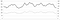A chart showing perfect correlation between two datasets. Correlation = 1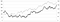A chart showing a strong correlation between two datasets. Correlation = 0.7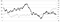A chart showing a strong negative correlation between two datasets. Correlation = -0.7

We must be extremely cautious with correlation. With financial data you will most likely have to calculate correlations of returns and not prices. If you’re interested in short-term relationships, go for returns’ correlations, otherwise, opt for prices if you’re in it for the long-term.

If you want to dig in deeper into Technical Analysis, you can read this article on a more advanced technique called Harmonic Patterns: Harmonic patterns in the FX market. How to detect them? Focus on ABCD’s

Written by